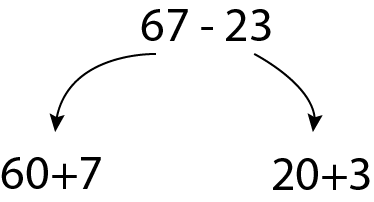# Mental Subtraction Strategies

Mental calculation doesn't mean performing the operations you do with paper and pencil in your head. In mental calculations, you need to use practical methods.

# 75-42

Instead of subtracting 42 directly from 75, we can subtract it in parts, 10 at a time.

75−10=65

65−10=55

55−10=45

45−10=35

35−2=33

You can count on your fingers how many times you subtracted 10; in total, we subtracted 42.

Alternatively, you can choose to do:

75 - 40 = 35

35 - 2 = 33

Of course, you will do these calculations mentally.

# Subtracting by breaking down into place values

##67 consists of 6 tens (60) and 7 ones, while 23 consists of 2 tens (20) and 3 ones.

Here, the problem is that I can subtract 30 from 70, but I can't subtract 6 from 4. What should we do?

# Cashier Method

The meaning of subtraction is to find the difference between two numbers, and the cashier method focuses directly on finding that difference.

When you shop at a grocery store or market, the cashier counts up from the amount you spent to find out how much change they need to give you. One meaning of subtraction is to find the difference. The cashier method directly finds this difference.

## 75 - 42 = ?

What is the difference between 75 and 42? That's the question we're answering.

Count from 42 to 75.
From 42 to 50 is 8,
and from 50 to 75 is 25,
so the total is 25 + 8 = 33.

# Maintaining the Difference in Subtraction

If there is a 2-year age difference between you and your older sibling, there was a 2-year difference 5 years ago, and there will be a 2-year difference 5 years from now. If you add or subtract the same number to both the minuend and the subtrahend, the difference doesn't change, meaning the result of your subtraction remains the same.

If you add or subtract the same number to both numbers ( subtrahend and minuend ) , you will preserve the difference.

## Example:

Instead of subtracting 42 from 75, We subtracted 2 from both numbers, so subtracting 40 from 73 is easier.

## Example;

Especially in numbers ending in zero, it makes subtraction easier;

Instead of subtracting 2376 from 5000, We subtracted 1 from both numbers, so we no longer needed to borrow 1 from the neighbor. We can subtract directly and more quickly

We added 7 to both numbers, subtracting 100 from one number is simpler.

We don't teach mental addition and subtraction just for it to be a lesson and then forgotten. We teach it so that you can use it in your daily life and in school. You will discover how simple and fun it is to use alternative methods as you practice. Even in seemingly complex calculations, you can use mental addition and subtraction (alternative ways).

You can even use it in schools for subtraction with very large numbers!

I subtracted 13 from both numbers ( subtrahend and  minuend )

I subtracted 5000 from both numbers.

I subtracted 400 from both numbers.

Consider 9004 as 9000 + 4.

Subtract 400 from 9000, which leaves 8600.

Combine the remaining numbers, 8600 and 4, to get 8604.

## So, 9004 - 400 = 8604.

In this subtraction, I followed my own order, but you can also subtract in your own sequence. It doesn't matter whether you first subtract 400 or 13, etc. You can start the process by subtracting any part that seems easy to you.